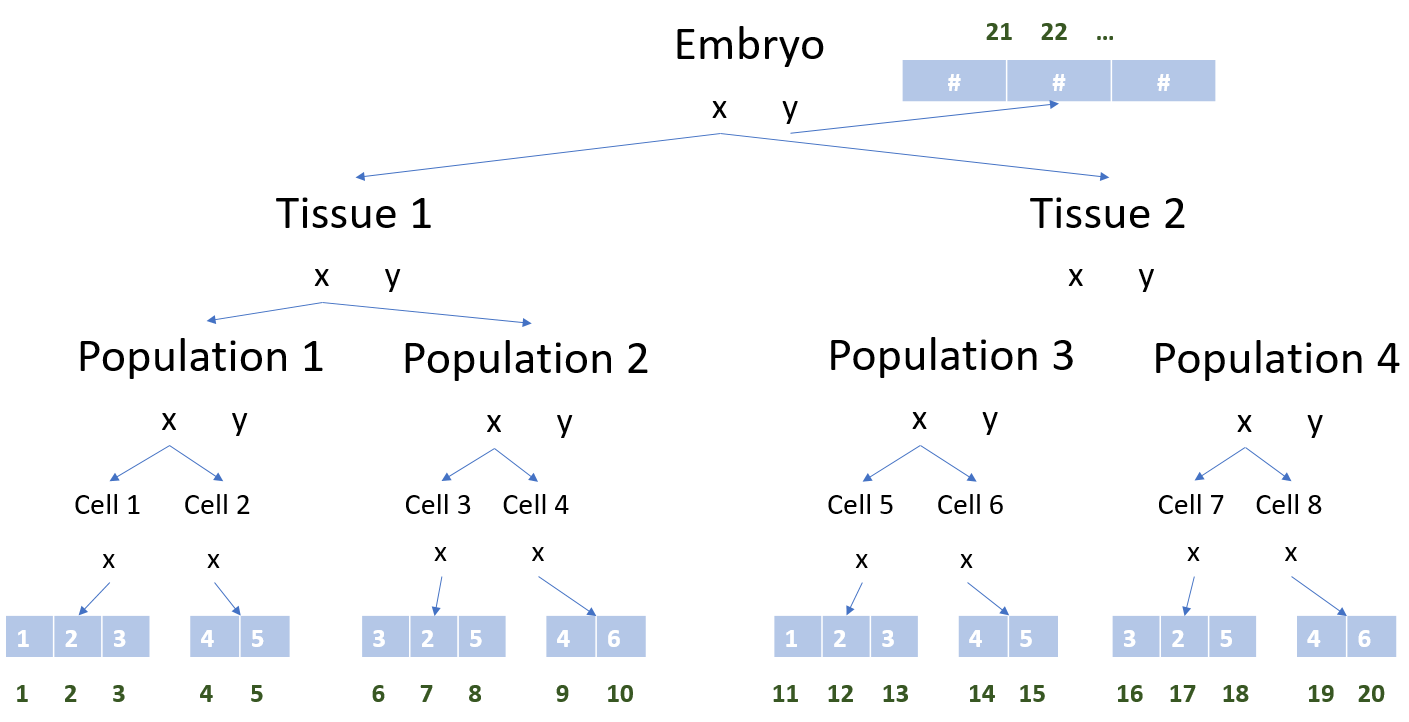# AbstractArray Libraries

## RecursiveArrayTools.jl: Arrays of Arrays and Even Deeper

Sometimes when one is creating a model, basic array types are not enough for expressing a complex concept. RecursiveArrayTools.jl gives many types, such as VectorOfArray and ArrayPartition, which allow for easily building nested array models in a way that conforms to the standard AbstractArray interface. While standard Vector{Array{Float64,N}} types may not be compatible with many equation solver libraries, these wrapped forms like VectorOfArray{Vector{Array{Float64,N}}} are, making it easy to use these more exotic array constructions.

Note that SciML's interfaces use RecursiveArrayTools.jl extensively, for example, with the timeseries solution types being AbstractVectorOfArray.

## LabelledArrays.jl: Named Variables in Arrays without Overhead

Sometimes you want to use a full domain-specific language like ModelingToolkit. Other times, you wish arrays just had a slightly nicer syntax. Don't you wish you could write the Lorenz equations like:

function lorenz_f(du,u,p,t)
du.x = p.σ*(u.y-u.x)
du.y = u.x*(p.ρ-u.z) - u.y
du.z = u.x*u.y - p.β*u.z
end

without losing any efficiency? LabelledArrays.jl provides the array types to do just that. All of the . accesses are resolved at compile-time so it's a zero-overhead interface.

Note

We recommend using ComponentArrays.jl for any instance where nested accesses is required, or where the . accesses need to be views to subsets of the array.

## MultiScaleArrays.jl: Multiscale Modeling to Compose with Equation SolversHow do you encode such real-world structures in a manner that is compatible with the SciML equation solver libraries? MultiScaleArrays.jl is an answer. MultiScaleArrays.jl gives a highly flexible interface for defining multi-level types which generates a corresponding interface as an AbstractArray. MultiScaleArrays.jl's flexibility includes the ease of resizing, allowing for models where the number of equations grows and shrinks as agents (cells) in the model divide and die.

Note

We recommend using ComponentArrays.jl instead in any instance where the resizing functionality is not used.

## ComponentArrays.jl: Arrays with Arbitrarily Nested Named Components

What if you had a set of arrays of arrays with names, but you wanted to represent them on a single contiguous vector so that linear algebra was as fast as possible, while retaining . named accesses with zero-overhead? This is what ComponentArrays.jl provides, and as such it is one of the top recommendations of AbstractArray types to be used. Multi-level definitions such as x = ComponentArray(a=5, b=[(a=20., b=0), (a=33., b=0), (a=44., b=3)], c=c) are common-place, and allow for accessing via x.b.a etc. without any performance loss. ComponentArrays are fully compatible with the SciML equation solvers, thus they can be used as initial conditions. Here's a demonstration of the Lorenz equation using ComponentArrays with Parameters.jl's @unpack:

using ComponentArrays
using DifferentialEquations
using Parameters: @unpack

tspan = (0.0, 20.0)

## Lorenz system
function lorenz!(D, u, p, t; f=0.0)
@unpack σ, ρ, β = p
@unpack x, y, z = u

D.x = σ*(y - x)
D.y = x*(ρ - z) - y - f
D.z = x*y - β*z
return nothing
end

lorenz_p = (σ=10.0, ρ=28.0, β=8/3)
lorenz_ic = ComponentArray(x=0.0, y=0.0, z=0.0)
lorenz_prob = ODEProblem(lorenz!, lorenz_ic, tspan, lorenz_p)

Is that beautiful? Yes it is.

## StaticArrays.jl: Statically-Defined Arrays

StaticArrays.jl is a library for statically-defined arrays. Because these arrays have type-level information for size, they recompile the solvers for every new size. They can be dramatically faster for small sizes (up to approximately size 10), but for larger equations they increase compile time with little to not benefit.

## CUDA.jl: NVIDIA CUDA-Based GPU Array Computations

CUDA.jl is the library for defining arrays which live on NVIDIA GPUs (CuArray). SciML's libraries will respect the GPU-ness of the inputs, i.e., if the input arrays live on the GPU then the operations will all take place on the GPU or else the libraries will error if it's unable to do so. Thus using CUDA.jl's CuArray is how one GPU-accelerates any computation with the SciML organization's libraries. Simply use a CuArray as the initial condition to an ODE solve or as the initial guess for a nonlinear solve and the whole solve will recompile to take place on the GPU.

## AMDGPU.jl: AMD-Based GPU Array Computations

AMDGPU.jl is the library for defining arrays which live on AMD GPUs (ROCArray). SciML's libraries will respect the GPU-ness of the inputs, i.e., if the input arrays live on the GPU then the operations will all take place on the GPU or else the libraries will error if it's unable to do so. Thus using AMDGPU.jl's ROCArray is how one GPU-accelerates any computation with the SciML organization's libraries. Simply use a ROCArray as the initial condition to an ODE solve or as the initial guess for a nonlinear solve and the whole solve will recompile to take place on the GPU.

## FillArrays.jl: Lazy Arrays

FillArrays.jl is a library for defining arrays with lazy values. For example, an O(1) representation of the identity matrix is given by Eye{Int}(5). FillArrays.jl is used extensively throughout the ecosystem to improve runtime and memory performance.

## BandedMatrices.jl: Fast Banded Matrices

Banded matrices show up in many equation solver contexts, such as the Jacobians of many partial differential equations. While the base SparseMatrixCSC sparse matrix type can represent such matices, BandedMatrices.jl is a specialized format specifically for BandedMatrices which can be used to greatly improve performance of operations on a banded matrix.

## BlockBandedMatrices.jl: Fast Block-Banded Matrices

Block banded matrices show up in many equation solver contexts, such as the Jacobians of many systems of partial differential equations. While the base SparseMatrixCSC sparse matrix type can represent such matices, BlockBandedMatrices.jl is a specialized format specifically for BlockBandedMatrices which can be used to greatly improve performance of operations on a block-banded matrix.